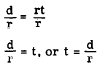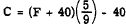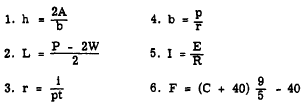Custom SearchSUBJECT OF A FORMULA Usually a formula is taken almost directly from the verbal rule or law. For instance, the perimeter of a rectangle is equal to twice the length plus twice the width. Where possible, letters are used as symbols for the words. Thus, P = 2L + 2W. A simple formula such as this is like a declarative sentence. The left half is the SUBJECT and all the rest is the predicate. The subject is P. It corresponds to the part of the verbal rule that reads "the perimeter of a rectangle." This subject is usually a single letter followed by the equality sign. All formulas are equations, but not all equations are formulas. Some distinctions between a formula and an ordinary equation are worthy of note. The equation may not have a subject, while the formula typically does. In the formula, the unknown quantity stands alone in the left-hand member. No computation is performed upon it, and it does not appear more than once. In the equation, on the other hand, the unknown quantity may appear once or more in either or both members, and computation may be performed with it or on it. We evaluate a formula by substituting for the literal numbers in the right member. An equation is solved by computation in either or both members until all that remains is an unknown in one member and a known quantity in the other. The solution of an equation usually requires a knowledge of algebraic principles, while the evaluation of a formula may ordinarily be accomplished with only a knowledge of arithmetic. SYMBOLS Letters that represent words have been standardized in many cases so that certain formulas may be written the same in various texts and reference books. However, to avoid any misunderstanding a short explanation often accompanies formulas as follows: A = hw, where         A = area in square units         h - height         w = width Subscripts and Primes In a formula in which two or more of the same kind of letters are being compared, it is desirable to make a distinction between them. In electronics, for example, a distinction between resistances may be indicated by Ra and Rb or R1 and R2. These small numbers or letters written to the right and below the R’s are called subscripts. Those shown here are read: R sub a, R sub b, R sub one, and R sub two. Primes are also used in the same manner to distinguish between quantities of the same kind. Primes are written to the right and above the letters, as in S’, S", and S"‘. They are read: S prime, S double prime, and S triple prime. CHANGING THE SUBJECT OF A FORMULA If values are given for all but one of its variables, a formula can be solved to obtain the value of that variable. The first step is usually the rearranging of the formula so that the unknown value is the subject-that is, a new formula is derived from the original. For example, the formula for linear motion-distance equals rate times time-is usually written d =rt Suppose that instead of the distance we wish to know the rate, r, or the time, t. We simply change the subject of the formula by the algebraic means developed in earlier chapters. Thus, in solving the formula for r, we divide both sides by t, with the following result:In words, this formula states that rate equals distance divided by time. Likewise, in solving for t, we have the following :In words, this formula states that time equals distance divided by rate. We have in effect two new formulas, the subject of one being rate and the subject of the other being time. They are related to the original formula because they were derived from it, but they are different in that they have different subjects. Practice problems. Derive new formulas from the following expressions with subjects as indicated:6. The modern formula for converting Fahrenheit temperatures to Celsius (centigrade) is. Express the formula for converting Celsius (centigrade) temperatures to Fahrenheit. Answers: